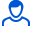Content: teorver_5561.doc (30.00 KB)

Positive responses: 0
Negative responses: 0

Refunds: 05561. x̅=111.2, n=20, σ=13. Given the standard deviation σ=13, the sample mean x̅в=111.2 and the sample size n=20 of a normally distributed feature of the general population. Find confidence intervals for estimating the general mean x̅g with a given reliability γ=0.99.
Detailed solution. Decorated in Microsoft Word 2003 (Quest decided to use the formula editor)
No feedback yet